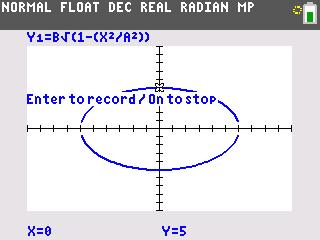Orbit Of Jupiter

This activity explores models for the elliptical orbit of Jupiter.
•TI-84 Plus CE
•TI-84 Plus C Silver Edition
• TI-84 Plus Silver Edition
• TI-84 PlusStudents will use the Transformational Graphing application to examine how the square root function is transformed on the coordinate plane.
•TI-84 Plus CE
•TI-84 Plus C Silver Edition
• TI-84 Plus Silver Edition
• TI-84 PlusComplex Numbers

Students calculate problems to determine the rules for adding, subtracting, multiplying, and dividing complex numbers.
•TI-84 Plus CE
•TI-84 Plus C Silver Edition
• TI-84 Plus Silver Edition
• TI-84 Plus••